International
Tables for
Crystallography
Volume B
Reciprocal space
Edited by U. Shmueli

International Tables for Crystallography (2006). Vol. B. ch. 4.5, pp. 475-476   | 1 | 2 |

## Section 4.5.2.6.3. Patterson functions

R. P. Millanea*

#### 4.5.2.6.3. Patterson functions

| top | pdf |

In fibre diffraction, the conventional Patterson function cannot be calculated since the individual structure-factor intensities are not available. However, MacGillavry & Bruins (1948)showed that the cylindrically averaged Patterson function can be calculated from fibre diffraction data. Consider the function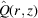defined by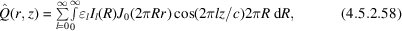where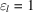forand 2 for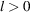, which can be calculated from the intensity distribution on a continuous fibre diffraction pattern. Using equations (4.5.2.7), (4.5.2.10), (4.5.2.17)and (4.5.2.58)shows thatis the cylindrical average of the Patterson function,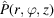, of one molecule, i.e.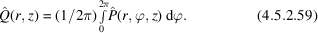Thesymbols onandindicate that these are Patterson functions of a single molecule, as distinct from the usual Patterson function of a crystal, which contains intermolecular interatomic vectors and is periodic with the same periodicity as the crystal.is periodic only along z and is therefore, strictly, a Patterson function along z and an autocorrelation function along x and y (Millane, 1990b). The cylindrically averaged Patterson contains information on interatomic separations along the axial direction and in the lateral plane, but no information on orientations of the vectors in the lateral plane.

For a polycrystalline system; consider the function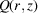given by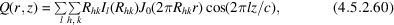where the sums are over all the overlapped reflections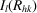on the diffraction pattern, given by equation (4.5.2.24). It is easily shown thatis related to the Patterson function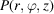by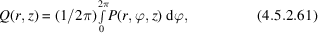where, in this case,is the usual Patterson function (expressed in cylindrical polar coordinates), i.e. it contains all intermolecular (both intra- and inter-unit cell) interatomic vectors and has the same translational symmetry as the unit cell. The cylindrically averaged Patterson function for polycrystalline fibres therefore contains the same information as it does for noncrystalline fibres (i.e. no angular information in the lateral plane), except that it also contains information on intermolecular separations.

Low resolution and cylindrical averaging, in addition to the usual difficulties with interpretation of Patterson functions, has resulted in the cylindrically averaged Patterson function not playing a major role in structure determination by fibre diffraction. However, information provided by the cylindrically averaged Patterson function has, in a number of instances, been a useful component in fibre diffraction analyses. A good review of the application of Patterson functions in fibre diffraction is given by Stubbs (1987). Removing data from the low-resolution part (or all) of the equator when calculating the cylindrically averaged Patterson function removes the strong vectors related to axially invariant (or cylindrically symmetric) parts of the map, and can aid interpretation (Namba et al., 1980; Stubbs, 1987). It is also important when calculating cylindrically averaged Patterson functions to use data only at a resolution that is appropriate to the size and spacings of features one is looking for (Stubbs, 1987).

Cylindrically averaged Patterson functions were used in early applications of fibre diffraction analysis (Franklin & Gosling, 1953; Franklin & Klug, 1955). The intermolecular peaks that usually dominate in a cylindrically averaged Patterson function can help to define the locations of multiple molecules in the unit cell. Depending on the space-group symmetry, it is sometimes possible to calculate the complete three-dimensional Patterson function (or certain projections of it). This comes about because of the equivalence of the amplitudes of overlapping reflections in some high-symmetry space groups. The intensity of each reflection can then be determined and a full three-dimensional Patterson map calculated (Alexeev et al., 1992). The only difficulty is that non-systematic overlaps are often present, although these are usually relatively few in number and the intensity can be apportioned equally amongst them, the resulting errors usually being small relative to the level of detail present in the Patterson map. For lower space-group symmetries, it may not be possible to calculate a three-dimensional Patterson map, but it may be possible to calculate certain projections of the map. For example, if the overlapped hk0 reflections have the same intensities, a projection of the Patterson map down the c axis can be calculated. Since such a projection is along the polymer axes, it gives the relative positions of the molecules in the ab plane. If the combined helix and space-group symmetry is high, an estimate of the electron density can be obtained by averaging appropriate copies of the three-dimensional Patterson function (Alexeev et al., 1992).

### ReferencesAlexeev, D. G., Lipanov, A. A. & Skuratovskii, I. Y. (1992). Patterson methods in fibre diffraction. Int. J. Biol. Macromol. 14, 139–144.Google ScholarFranklin, R. E. & Gosling, R. G. (1953). The structure of sodium thymonucleate fibres. II. The cylindrically symmetrical Patterson function. Acta Cryst. 6, 678–685.Google ScholarFranklin, R. E. & Klug, A. (1955). The splitting of layer lines in X-ray fibre diagrams of helical structures: application to tobacco mosaic virus. Acta Cryst. 8, 777–780.Google ScholarMacGillavry, C. H. & Bruins, E. M. (1948). On the Patterson transforms of fibre diagrams. Acta Cryst. 1, 156–158.Google ScholarMillane, R. P. (1990b). Phase retrieval in crystallography and optics. J. Opt. Soc. Am. A, 7, 394–411.Google ScholarNamba, K., Wakabayashi, K. & Mitsui, T. (1980). X-ray structure analysis of the thin filament of crab striated muscle in the rigor state. J. Mol. Biol. 138, 1–26.Google ScholarStubbs, G. (1987). The Patterson function in fibre diffraction. In Patterson and Pattersons, edited by J. P. Glusker, B. K. Patterson & M. Rossi, pp. 548–557. Oxford University Press.Google Scholar